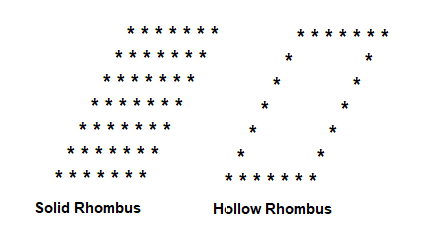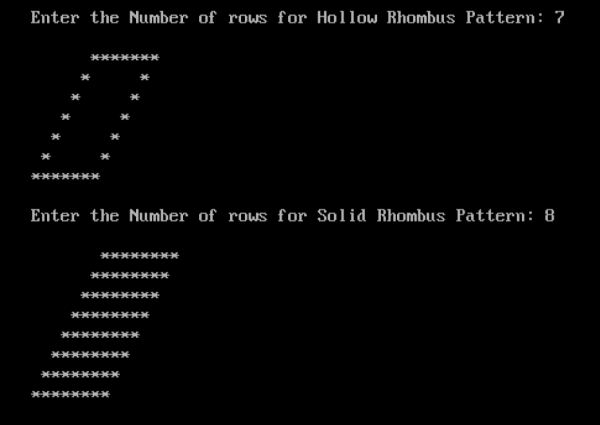# Program to print solid and hollow rhombus patterns in C

## Program Description

Print the Solid and Hollow Rhombus Patterns as show below## Algorithm

For Hollow Rhombus −

Accept the Number of Rows for Hollow Rhombus from the User
Create a Hollow Rhombus containing the same number of Rows specified by the User.
Print the first row containing the number of stars same as the number of rows.
Print the second row containing the first and last star as show in the output and leave the spaces between first and the last star.
Do the same till you reach the last Row.
Print the last row containing the number of stars same as the number of rows.

For Solid Rhombus −

Accept the Number of Rows for Solid Rhombus from the User
Create a Solid Rhombus containing the same number of Rows specified by the User.
Print the first row containing the number of stars same as the number of rows.
Do the same till you reach the last Row.

## Example

/* Program to print Hollow and Solid Rhombus star pattern */
#include <stdio.h>
int main() {
int r, c, rows; //Hollow Rhombus
int r1,c1, rows1; //Solid Rhombus
clrscr();
printf("Enter the Number of rows for Hollow Rhombus Pattern: ");
scanf("%d", &rows);
printf("");
for(r=1; r<=rows; r++){
for(c=1; c<=rows-r; c++){
printf(" ");
}
for(c=1; c<=rows; c++){
if(r==1 || r==rows || c==1 || c==rows)
printf("*");
else
printf(" ");
}
printf("");
}
printf("");
printf("Enter the Number of rows for Solid Rhombus Pattern: ");
scanf("%d", &rows1);
printf("");
for (r1=1; r1<=rows1; r1++){
for (c1=1; c1<=rows1-r1;c1++){
printf(" ");
}
for (c1=1; c1<=rows1; c1++){
printf("*");
}
printf("");
}
getch();
return 0;
}

## Output